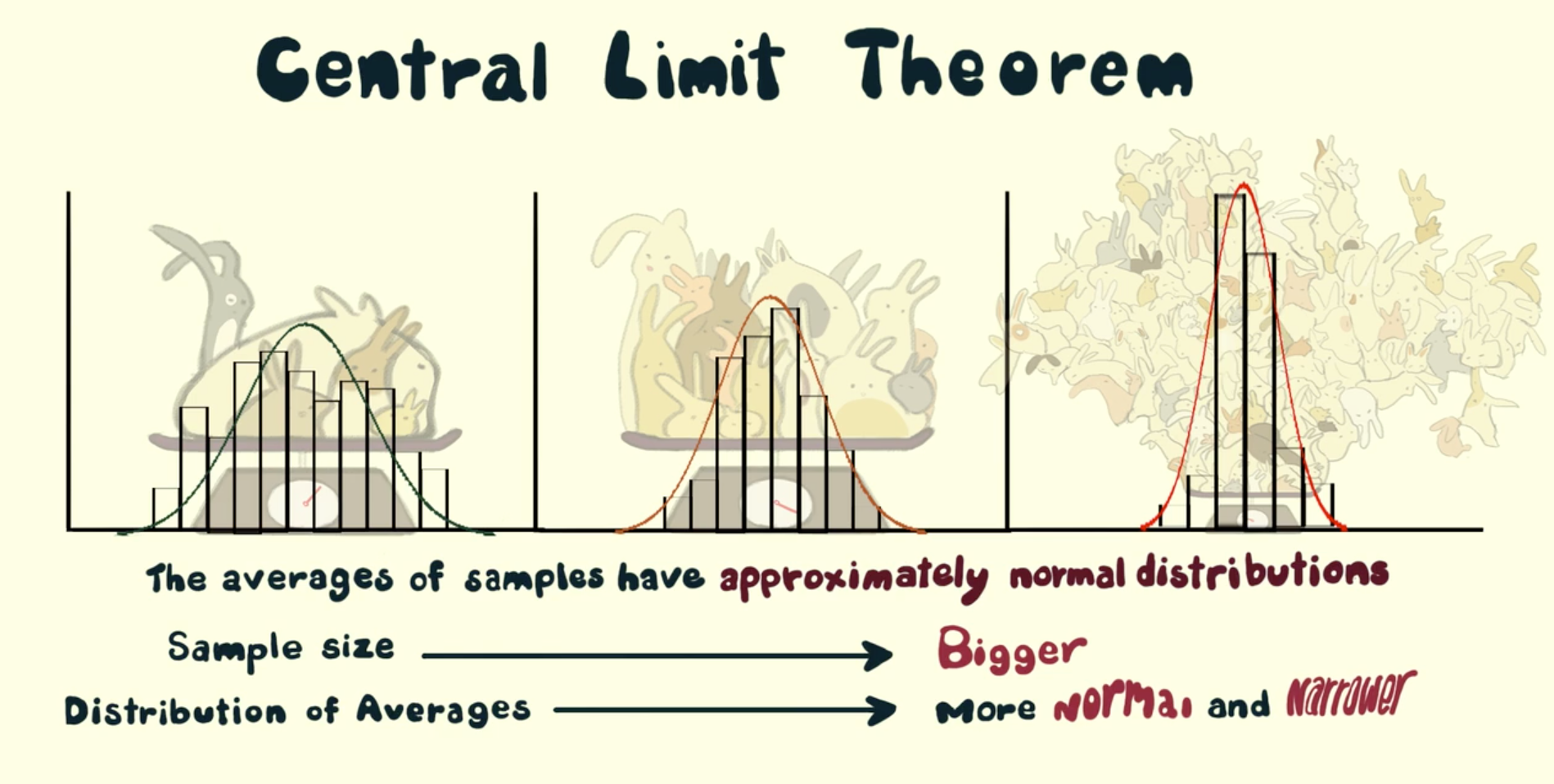# 5.3 The Central Limit TheoremHarrison BurnsideJosh Argo

Brianna Bukowski

## Definition

The Central Limit Theorem is often tested on free response questions dealing with quantitative data (means). Now, you cannot assume that the sample is normally distributed. The question must either explicitly state so, or you have to follow the central limit theorem.
The Central Limit Theorem states that if n (the sample size) is large, the sampling distribution is normal. The larger n is, the more normal the sample is.
So, what is large? If n is greater or equal to 30, it is considered large. Thus, if the same size is greater than or equal to 30, it is approximately normally distributed.
Also, the samples must all be independent of each other. One way to assume this is if the sample size is 10% or less of the population.
Another aspect to check is that the sample is a simple random sample.
So, for a quantitative sample to be normally distributed according to the central limit theorem, it must:
• Be independent
• Be a random sample
• Have an n that is greater than 30

## Example

Use the Central Limit Theorem when calculating the probability about a mean or average.
For example, if a question asks for the probability about the mean size of fish, you cannot assume that the sample of fish, say in a pond, is greater than 30 unless the problem states so.
In this question, reasoning might include that since the sample of fish is 40, which is greater than 30, it is approximately normal. This is because according to the central limit theorem, if n is large, then the sample is approximately normally distributed. Additionally, you must state that the samples are independent of each other.  This is true for both quantitative and categorical data (and the statement is the same).
You must explicitly state that you assumed the sample is normally distributed because of the central limit theorem.
The picture below shows what happens as you increase your sample size. The smallest triangle is a sample size of 5 and the tallest is a sample size of 100.Source: SurgeForce Demo

### Bigger is Better!

With sampling distributions, the larger the sample size, the less spread the curve is going to have. This is because the larger a sample is, the more the sampling distribution is going to hone in on the true population parameter
Think of this...if you flip a coin 6 times, the proportion of heads you get is likely to be greater than or less than 0.5 (the data is somewhat skewed). However, once you flip the coin 50, 100, 1000 times, it is very unlikely that the proportion of heads is going to be much different from the true population proportion, which we know is 0.5.
Due to this concept, the larger the sample size, the better.   A large sample size allows us to hone in on the population parameter (either 𝝁 or 𝝆), which is EXACTLY what we are after when using a sampling distribution.

##### 🔍 Are you ready for college apps?
Take this quiz and find out!
Start Quiz
#####FREE AP stats Survival Pack + Cram Chart PDF
Browse Study Guides By Unit
📆
Big Reviews: Finals & Exam Prep
✏️
Blogs
✍️
Free Response Questions (FRQs)
👆
Unit 1: Exploring One-Variable Data
✌️
Unit 2: Exploring Two-Variable Data
🔎
Unit 3: Collecting Data
🎲
Unit 4: Probability, Random Variables, and Probability Distributions
⚖️
Unit 6: Inference for Categorical Data: Proportions
😼
Unit 7: Inference for Qualitative Data: Means
✳️
Unit 8: Inference for Categorical Data: Chi-Square
📈
Unit 9: Inference for Quantitative Data: Slopes
Thousands of students are studying with us for the AP Statistics exam.
#####